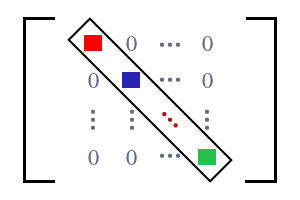# Diagonal Matrix

A matrix that consists of zeros as entries (or elements) outside the main diagonal is called a diagonal matrix.

## IntroductionSquare matrices are appeared with zeros. In a special case, a square matrix contains zero as non-diagonal elements but it contains elements only on principal diagonal. Due to having elements on leading diagonal and having zeros as non-diagonal elements, the square matrix is recognized as a diagonal matrix.

The matrix is having elements ${e}_{1⁣1},{e}_{2⁣2},{e}_{3⁣3},\dots {e}_{m⁣m}$ only on principal diagonal but observe the elements on non-diagonal areas. All are zero elements at non-diagonal areas. Therefore, this type of matrix is called a diagonal matrix. The diagonal elements can be either equal or unequal elements.

It is simply expressed as

## Example

$D$ is a square matrix of order $5×5$. It is having $25$ element in five rows and five columns.

The matrix $D$ is having two types of elements. One type of elements are nonzero elements and remaining all are zeros. Nonzero elements ($1,–5,7,3$ and $9$) are placed on the leading diagonal and remaining non-diagonal elements are zeros. Therefore, the matrix $D$ is known as a diagonal matrix.

The diagonal matrix $D$ is written in simple form

Latest Math Topics
Jun 26, 2023
Jun 23, 2023

###### Math Questions

The math problems with solutions to learn how to solve a problem.

Learn solutions

Practice now

###### Math Videos

The math videos tutorials with visual graphics to learn every concept.

Watch now

###### Subscribe us

Get the latest math updates from the Math Doubts by subscribing us.